7 篇文章 8 订阅

# 钢管切割问题（一维）

## 题目1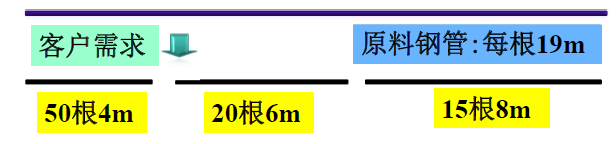### 分析

1、下料最省的标准（也就是最后的目标函数）

• 原料钢管剩余总余量最小;
• 所用原料钢管总根数最少.

2、由于采用不同切割模式太多, 会增加生产和管理成本,所以要规定切割模式不能超过几种，即切割模式自己设定为固定的几种。

3、按照客户需要在一根原料钢管上安排切割的某种排列组合，合理切割模式的余料应小于客户需要钢管的最小尺寸。

4、约束条件：

• 需求约束
• 原料约束
• 整数约束

### 求解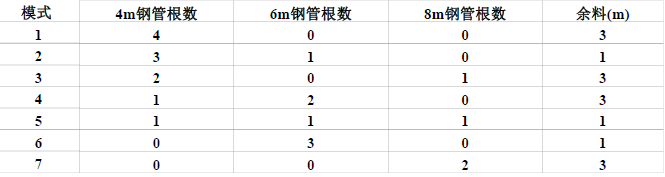#### 思路1——总余量最少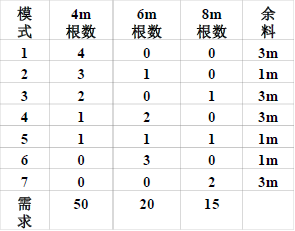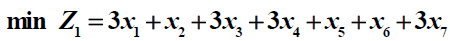• 需求约束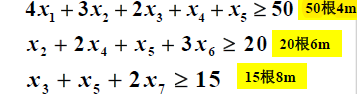• 整数约束
xi均为整数

Lingo代码

model:
sets:
endsets
data:
f=3,1,3,3,1,1,3;b=50,20,15;
A=4,3,2,1,1,0,0
0,1,0,2,1,3,0
0,0,1,0,1,1,2;
enddata
min=@sum(schema(i):(f(i)*x(i)));
@for(pipe(i):(@sum(schema(j):A(i,j)*x(j)))>=b(i));
@for(schema(i):@gin(x(i)));
end


#### 思路2——总根数最少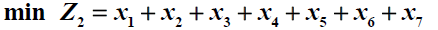## 题目2

### 求解

r1i, r2i, r3i, r4i是第i 种切割模式下, 每根原料钢管生产4m、5m、6m和8m长的钢管的数量.

• 需求约束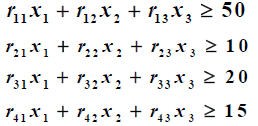• 原料约束（切割模式合理）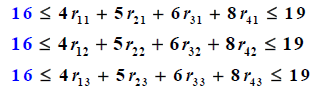• 缩小可行域约束（根据第一问的规律得出）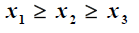• 整数约束
xi,rij均为整数

Lingo代码

model:
sets:
endsets
data:
b=50,10,20,15;c=4,5,6,8;
enddata
min=@sum(schema(i):x(i));
@for(pipe(i):(@sum(schema(j):r(i,j)*x(j)))>=b(i));
@for(schema(j):(@sum(pipe(i):r(i,j)*c(i)))<19);
@for(schema(j):(@sum(pipe(i):r(i,j)*c(i)))>=16);
@for(schema(i):@gin(x(i)));
@for(schema(j):@for(pipe(i):@gin(r(i,j))));
x(1)>=x(2);
x(2)>=x(3);
end


# 易拉罐下料（二维）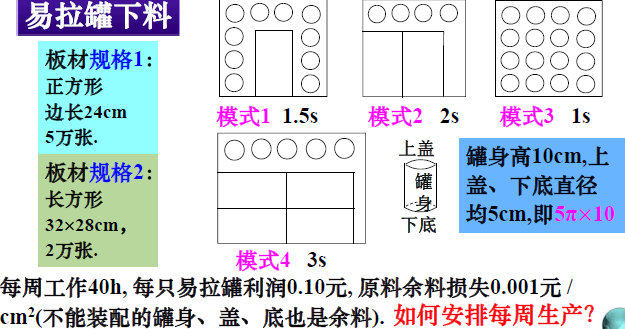## 求解

xi ~ 按照第i 种模式的生产张数(i=1,2,3,4)；
y1 ~ 一周生产的易拉罐个数；
y2 ~ 不配套的罐身个数；
y3 ~ 不配套的底、盖个数.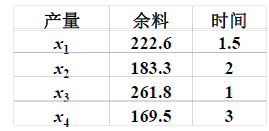max=0.1y1-0.001(222.6x1+183.3x2+261.8x3+169.5y2)

• 时间约束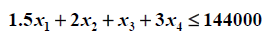• 原料约束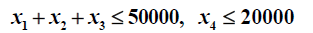• 配套约束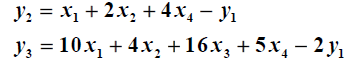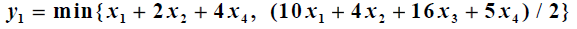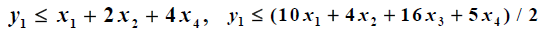# 切割模式相关软件

CutMasterCutLogic 2D# 附三维问题：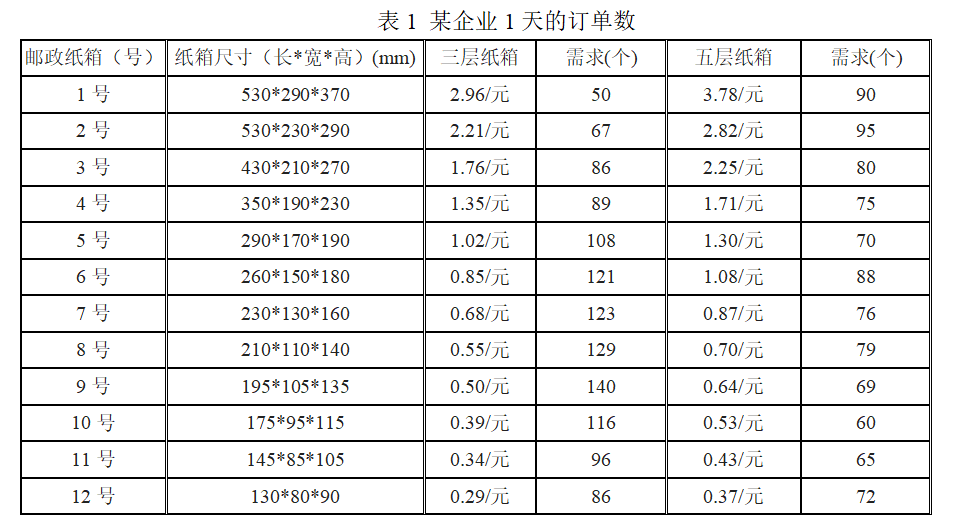08-2005-161万+
04-191万+
07-14
08-21
04-068102
04-192834
04-158010
07-06
08-147086
03-23573
04-07388
04-109001点击重新获取扫码支付余额充值